• ## Matlab读写.mat数据

万次阅读 多人点赞 2018-06-08 08:57:44
.mat文件的方便之处在于，它可以连同数据的变量名一同保存下来（这一点在程序调试的过程中很方便），并且不需要控制数据的存储格式，matlab会自动保存并区分我们所存储的内容。利用load和save指令可以实现对.mat文件的读写。

（1）利用save指令写.mat文件

首先在Editor内建立一些示例变量，如下所示：

A = [1 2 3; 4 5 6; 7 8 9];
B = [1 0; 0 1];
C = 'XYZ';
D = 256;
此时运行matlab，可以在Workspace内看到这些变量，如下图所示：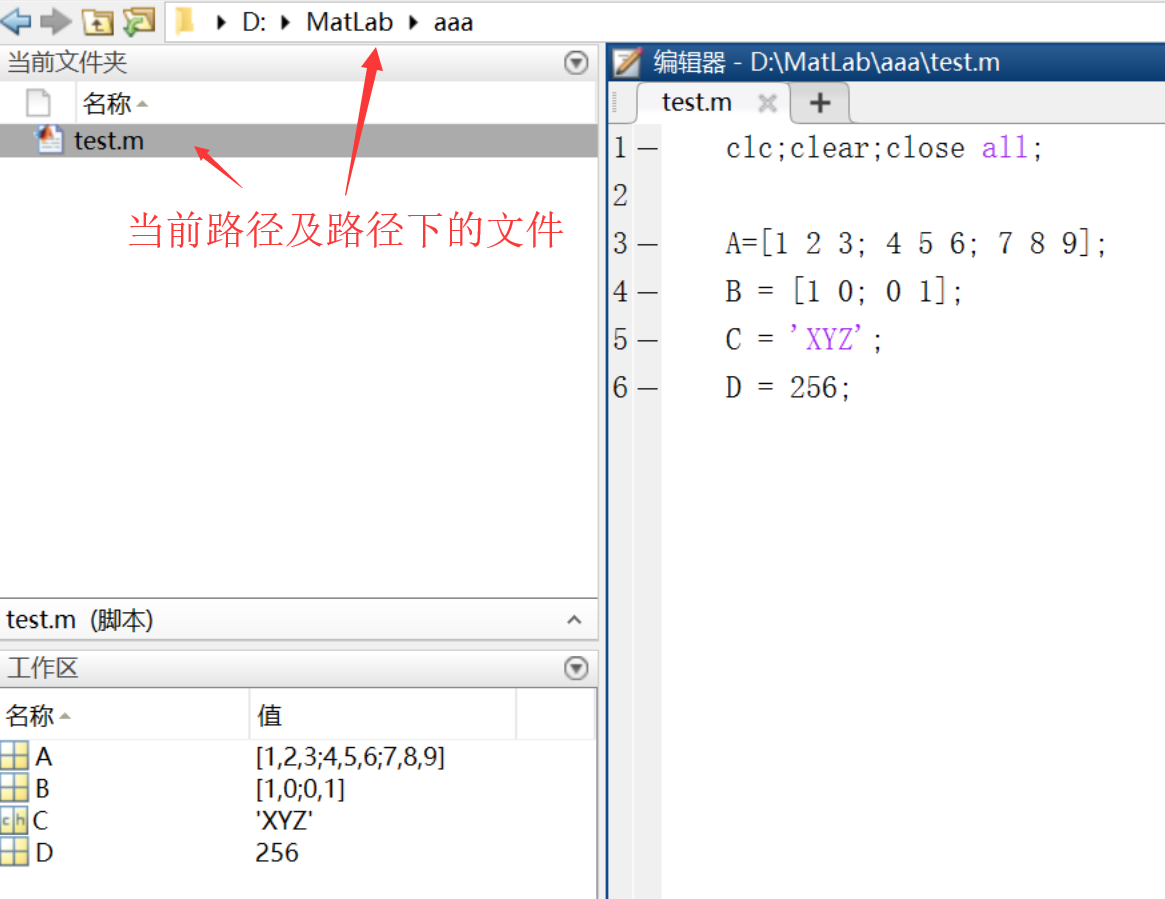此时添加下述程序：（可以在Command Window内写，也可以在Editor内写）

save matdata;
则名称为matdata.mat的文件保存在当前默认的路径下了，如下图所示：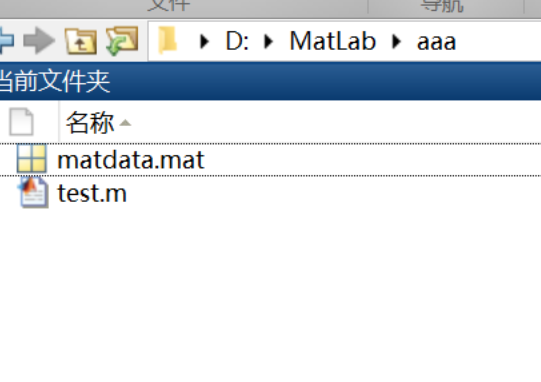此时Workspace内所有的数据连同他们的变量名称都保存在matdata.mat文件内，

如果只想保存指定的数据，则可以使用如下命令：

save mydata A D;
此时被指定的数据A和D被保存在mydata.mat文件内，保存路径也是当前默认的，如下图所示：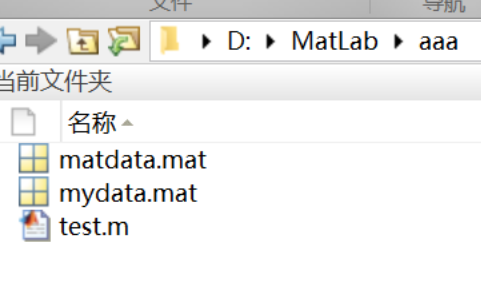若要保存全部数据中的某一部分，则可以调用如下命令：

save ('filename', 'dataname');
其中filename是.mat的名称；dataname是需要保存的数据的名字。

利用上面的指令就读入了mydata.mat内的数据，可以看到此时的Workspace内只有A和D的数据，如下图所示：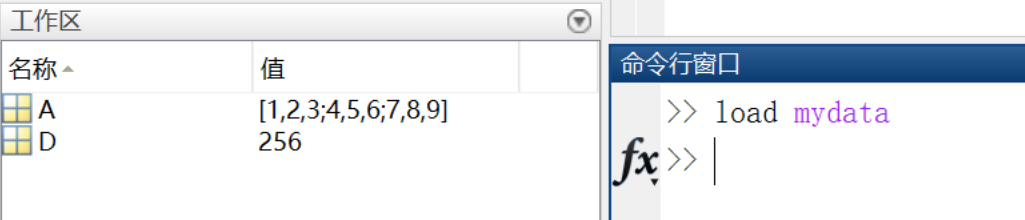展开全文• 以http://ufldl.stanford.edu/housenumbers/上的mat数据集为例需要注意以下几点从mat提取出来的数据以字典的形式保存，所以需要提取字典的key和valueimport numpy as npimport osfrom PIL import Imageimport ...
以http://ufldl.stanford.edu/housenumbers/上的mat数据集为例需要注意以下几点从mat提取出来的数据以字典的形式保存，所以需要提取字典的key和valueimport numpy as npimport osfrom PIL import Imageimport matplotlib.pyplot as pltimport scipy.io as sciodata_train=scio.loadmat(r'data/train_32x32.mat')#print(data_train)#取出需要的数据矩阵data_train_data=data_train.get('X')#print(data_train_data)#输出字典里的X#data_train_label=data_train.get('y')#提取标签#print(len(data_train_label))for i in range(10):plt.subplot(2,5,i+1)#plt.title(data_train_label[i])#print("标签"+str(data_train_label[i]))plt.imshow(data_train_data[...,i])#print("数据"+str(data_train_data[...,i])+"------")plt.axis('off')plt.savefig('picture/1.png')plt.show()输出结果批量处理将mat文件里的所有图片进行输出并保存在不同的画布中import numpy as npimport osfrom PIL import Imageimport matplotlib.pyplot as pltimport scipy.io as sciodata_train=scio.loadmat(r'data/train_32x32.mat')print(data_train)#取出需要的数据矩阵data_train_data=data_train.get('X')print(data_train_data)#输出字典里的Xdata_train_label=data_train.get('y')#提取标签print(len(data_train_label))for i in range(10):#如果要全部输出，这里应该改成73256#plt.subplot(1,1,i+1)#plt.figure()plt.title(data_train_label[i])print("标签"+str(data_train_label[i]))plt.imshow(data_train_data[...,i])print("数据"+str(data_train_data[...,i])+"------")plt.axis('off')plt.savefig('picture/' +str(i)+'.png')plt.show()#此函数读取特定文件夹下的mat格式图像，返回图片所在路径的列表def get_imlist(path):for f in os.listdir(path):if f.endswith('.mat'):return os.path.join(path,f)#路径加起来#print(os.path.join(path,f))c=get_imlist(path)print(c)d=len(path)print(d)输出结果展开全文• flickr.mat数据集，可以用于网络表示学习的数据集，论文中常用flickr
• opencv中Mat数据结构1 Mat类结构1.1 数据部分1.2 函数部分1.2.1 构造函数和析构函数1.2.2 运算符重载1.2.3 数据访问函数1.2.4 其它常用函数2 矩阵元素数据存储方式2.1 存储方式2.2 矩阵数据的读写（不用opencv） ...
opencv中Mat数据结构1 Mat类结构1.1 数据部分1.2 函数部分1.2.1 构造函数和析构函数1.2.2 运算符重载1.2.3 数据访问函数1.2.4 其它常用函数2 矩阵元素数据存储方式2.1 存储方式2.2 矩阵数据的读写（不用opencv）
1 Mat类结构

Mat是matrix矩阵的简写，Mat类中数据部分主要分为两个部分：头和数据指针。头中定义一些矩阵的行数、列数、通道数、维度等信息；数据指针中定义uchar* data的数据地址。函数部分主要包括大量构造函数、大量模版函数以及一些非模版函数。
我们知道在c++中类实例化的对象中，真正拥有的是数据部分。

1.1 数据部分
int flags; //signature
int dims; //维数，灰度图像二维，rgb图像三维
int rows, cols; //行数和列数，这里说的是逻辑上的矩阵的行数和列数，而不是物理存储时
uchar* data; // 矩阵元素数据的首地址，类型是uchar*，在c/c++中通常最小的数据处理大小是一个字节

//图像ROI的辅助指针
uchar* datastart;
uchar* dataend;
uchar* datalimit;

MSize size; //一行含有的字节数，MSize和MStep是定义的结构体
MStep step;
//...

1.2 函数部分

1.2.1 构造函数和析构函数

Mat();
Mat(int rows, int cols, int type);
Mat(Size size, int type);
Mat(int rows, int cols, int type, const Scalar& s);
Mat(Size size, int type, const Scalar& s);
Mat(int ndims, const int* sizes, int type);
Mat(int ndims, const int* sizes, int type, const Scalar& s);

Mat(const Mat& m);

Mat(int rows, int cols, int type, void* data, size_t step=AUTO_STEP);
Mat(Size size, int type, void* data, size_t step=AUTO_STEP);
Mat(int ndims, const int* sizes, int type, void* data, const size_t* steps=0);

Mat(const Mat& m, const Range& rowRange, const Range& colRange=Range::all());
Mat(const Mat& m, const Rect& roi);
Mat(const Mat& m, const Range* ranges);

Mat(const IplImage* img, bool copyData=false);
//...
~Mat();


1.2.2 运算符重载

Mat& operator = (const Mat& m); //赋值运算符重载，做的是浅拷贝
Mat& operator = (const MatExpr& expr);
Mat& operator = (const Scalar& s);

Mat operator()( Range rowRange, Range colRange ) const;
Mat operator()( const Rect& roi ) const;
Mat operator()( const Range* ranges ) const;

CV_EXPORTS MatExpr operator * (const Mat& a, const Mat& b);//矩阵内积

operator CvMat() const;
operator CvMatND() const;
operator IplImage() const;

template<typename _Tp> operator vector<_Tp>() const;
template<typename _Tp, int n> operator Vec<_Tp, n>() const;
template<typename _Tp, int m, int n> operator Matx<_Tp, m, n>() const;


1.2.3 数据访问函数

ptr函数


// 返回矩阵元素指针
uchar* ptr(const int* idx);
const uchar* ptr(const int* idx) const;

template<typename _Tp> _Tp* ptr(int i0=0);
template<typename _Tp> const _Tp* ptr(int i0=0) const;

template<typename _Tp> _Tp* ptr(int i0, int i1);
template<typename _Tp> const _Tp* ptr(int i0, int i1) const;

template<typename _Tp> _Tp* ptr(int i0, int i1, int i2);
template<typename _Tp> const _Tp* ptr(int i0, int i1, int i2) const;

template<typename _Tp> _Tp* ptr(const int* idx);
template<typename _Tp> const _Tp* ptr(const int* idx) const;

template<typename _Tp, int n> _Tp* ptr(const Vec<int, n>& idx);
template<typename _Tp, int n> const _Tp* ptr(const Vec<int, n>& idx) const;


at函数

template<typename _Tp> _Tp& at(int i0=0);
template<typename _Tp> const _Tp& at(int i0=0) const;

template<typename _Tp> _Tp& at(int i0, int i1);
template<typename _Tp> const _Tp& at(int i0, int i1) const;

template<typename _Tp> _Tp& at(int i0, int i1, int i2);
template<typename _Tp> const _Tp& at(int i0, int i1, int i2) const;

template<typename _Tp> _Tp& at(const int* idx);
template<typename _Tp> const _Tp& at(const int* idx) const;

template<typename _Tp, int n> _Tp& at(const Vec<int, n>& idx);
template<typename _Tp, int n> const _Tp& at(const Vec<int, n>& idx) const;

//! special versions for 2D arrays (especially convenient for referencing image pixels)
template<typename _Tp> _Tp& at(Point pt);
template<typename _Tp> const _Tp& at(Point pt) const;


迭代器函数

template<typename _Tp> MatIterator_<_Tp> begin();
template<typename _Tp> MatIterator_<_Tp> end();
template<typename _Tp> MatConstIterator_<_Tp> begin() const;
template<typename _Tp> MatConstIterator_<_Tp> end() const;


1.2.4 其它常用函数

matlab风格的初始化函数，静态函数
矩阵运算
返回通道数等
深拷贝函数

//matlab风格的初始化函数，静态函数
static MatExpr zeros(int rows, int cols, int type);
static MatExpr zeros(Size size, int type);
static MatExpr ones(int rows, int cols, int type);
static MatExpr ones(Size size, int type);
static MatExpr eye(int rows, int cols, int type);
static MatExpr eye(Size size, int type);

//矩阵运算
Mat cross(InputArray m) const; //一维向量的叉乘，即外积
double dot(InputArray m) const; //一维向量内积，结果是一个数
MatExpr mul(InputArray m, double scale=1) const; //矩阵对应元素的乘积
//两个矩阵的内积有重载的*可以实现 即 C=A*B，A的行数要与B的列数一致
MatExpr inv(int method=DECOMP_LU) const; //矩阵的逆矩阵
MatExpr t() const; //矩阵转置

//
size_t total() const;//返回图像中像素点总数量=rows*cols
int type() const; //返回类型CV_8UC1 CV_16SC1 CV_32FC3等
int depth() const;
int channels() const; //返回通道数
size_t elemSize() const; //返回像素元素的大小以字节的方式，包括通道。
bool isContinuous() const; //判断数据存储是否连续
void create(int rows, int cols, int type); //如果之前矩阵没有创建数据，可以创建

//深拷贝函数
Mat clone() const;
void copyTo( OutputArray m ) const;
void copyTo( OutputArray m, InputArray mask ) const;
//...

2 矩阵元素数据存储方式
2.1 存储方式

uchar data;对应的是数据的首元素存储地址。
数据存储时，逐步扫描行，在内存中按照读的顺序存储。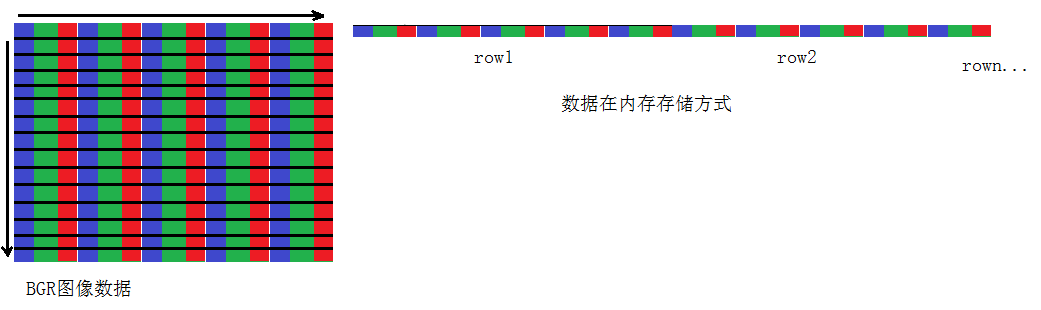根据数据的存储方式，可知要获取一个图像或矩阵的元素的数据，有几个关键点：

获得存储数据的首地址，即uchar* data;
获得图像或矩阵的通道数，是单通道（灰度图像）还是三通道（RGB）图像，即

Mat src;
int src.channels();

获得每个元素的字节大小。

src.elemSize(); //返回元素所有通道一共的字节大小。如CV_8UC3，返回的就是1*3=3
src.elemSize1(); //返回元素单通道的字节大小。如CV_8UC3，返回的就是1

2.2 矩阵数据的读写（不用opencv）
//data 是数据存储的首地址，
//channels是图像的通道数，
//elemSize是每个元素单通道中的字节数
// rows 行数
// cols 列数
void work(uchar* data,int channels,int elemSize，int rows,int cols)
{
for (int i=0;i<rows;i++)
{
uchar* p = data + cols*channels*elemSize*i; //获得i行的首地址
for (int j=0;j<cols;j++)
{
uchar* pj = p+j*channels*elemSize; //获得第j列的首地址
//do something
}
}
}



展开全文opencv
• Android 开发过程中为了实现代码的高效性，通常要调用本地c++代码，JNI是java语言提供的和c/c++相互...c++部分新建Mat指针指向java传入的内存区域，将处理后的结果图像的Mat数据复制到这块内存区域，这样java中的创建的
• usps数据库为标准手写数据库，此为mat数据文件，含训练集和测试
• Mat数据结构 一开始OpenCV是基于C语言的，在比较早的教材例如《学习OpenCV》中，讲解的存储图像的数据结构还是IplImage，这样需要手动管理内存。现在存储图像的基本数据结构是Mat。 Mat是opencv中保存图像数据...

Mat数据结构

一开始OpenCV是基于C语言的，在比较早的教材例如《学习OpenCV》中，讲解的存储图像的数据结构还是IplImage，这样需要手动管理内存。现在存储图像的基本数据结构是Mat。
Mat是opencv中保存图像数据的基本容器。其定义如下:
class CV_EXPORTS Mat
{
public:
// ... a lot of methods ...
...

/*! includes several bit-fields:
- the magic signature
- continuity flag
- depth
- number of channels
*/
int flags;
//! the array dimensionality, >= 2
int dims;
//! the number of rows and columns or (-1, -1) when the array has more than 2 dimensions
int rows, cols;
//! pointer to the data
uchar* data;

//! pointer to the reference counter;
// when array points to user-allocated data, the pointer is NULL
int* refcount;

// other members
...
};

Mat类可以表示n维的单通道或多通道数组，它可以存储实数/复数的向量和矩阵，单色或彩色图像等。向量MM的布局是由数组M.step[]M.step[]决定的，元素(i0,...,iM.dims−1)(i0,...,iM.dims−1)的地址为（其中0≤ik<M.size[k]0≤ik<M.size[k])：

Mat对象的数据布局和CvMat、Numpy等兼容，实际上它和以step(strides)方式计算像素地址方式的数据结构兼容。
在上面的数据结构可以看出，Mat数据结构中指针信息可以共享，即矩阵头信息独立，矩阵数据可以共享，使用引用计数器，类似智能指针。这样用户使用时，用户可以分配Mat的头信息，共享数据信息，并在原地处理信息，这样可以极大的节省内存。

创建Mat对象

1、使用构造函数Mat(nrows,
ncols, type[, fillValue])或者create(nrows,
ncols, type)
这样可以创建一个nrows行，ncol列的矩阵，类型为type。例如CV_8UC1表示8位单通道，
CV_32FC2表示双通道32位floating-point双通道。
// make a 7x7 complex matrix filled with 1+3j.
Mat M(7,7,CV_32FC2,Scalar(1,3));
// and now turn M to a 100x60 15-channel 8-bit matrix.
// The old content will be deallocated
M.create(100,60,CV_8UC(15));
对于type，格式为CV_位数+数值类型+C通道数，例如：
CV_8UC1表示：单通道阵列，8bit无符号整数
CV_8US2表示：2通道阵列，8bit有符号整数)
2、创建多维矩阵

// create a 100x100x100 8-bit array
int sz[] = {100, 100, 100};
Mat bigCube(3, sz, CV_8U, Scalar::all(0));

3、使用拷贝构造函数或赋值操作符时，只是创建了矩阵头，共享了矩阵信息，时间复杂度为O(1)。Mat::clone()函数是深拷贝，拷贝了Mat的所有信息。

4、只创建信息头部分，时间复杂度为O(1)，可以使用这个特征Mat局部信息：

// add the 5-th row, multiplied by 3 to the 3rd row
M.row(3) = M.row(3) + M.row(5)*3;

// now copy the 7-th column to the 1-st column
// M.col(1) = M.col(7); // this will not work
Mat M1 = M.col(1);
M.col(7).copyTo(M1);

// create a new 320x240 image
Mat img(Size(320,240),CV_8UC3);
// select a ROI
Mat roi(img, Rect(10,10,100,100));
// fill the ROI with (0,255,0) (which is green in RGB space);
// the original 320x240 image will be modified
roi = Scalar(0,255,0);

可以创建ROI（Region of interest）区域

Mat A = Mat::eye(10, 10, CV_32S);
// extracts A columns, 1 (inclusive) to 3 (exclusive).
Mat B = A(Range::all(), Range(1, 3));
// extracts B rows, 5 (inclusive) to 9 (exclusive).
// that is, C ~ A(Range(5, 9), Range(1, 3))
Mat C = B(Range(5, 9), Range::all());
Size size; Point ofs;
C.locateROI(size, ofs);
// size will be (width=10,height=10) and the ofs will be (x=1, y=5)

6、使用Mat::eye(), Mat::zeros(), Mat::ones()创建矩阵；或使用Mat_()
Mat E = Mat::ones(2, 2, CV_32F);
Mat O = (Mat_<float>(3, 3) << 1, 2, 3, 4, 5, 6, 7, 8, 9);

矩阵运算

Mat支持矩阵运算。A、B表示Mat类型对象，s表示标量，alpha表示实数，支持以下运算：
** 加：A+B, A-B, A+s, s+B，-A
** 缩放：A*alpha对应像素相乘/除：A.mul(B)，A/B, alpha/A。这里要求A、B大小相等，数据类型通道数一致。转置：A.t()，相当于A^T
** 求逆和伪逆矩阵。A.inv([method])宝石求逆，A.inv([method])*B表示求X，其中X满足AX=B逻辑位操作 A & B, ~A内积：A.cross(B), A.dot(B)，表示对应像素相乘，求和。

常用接口

1、C++:
size_t Mat::total() const
返回像素总数
2、C++:
int Mat::depth() const
返回矩阵type类型对应的数值。
3、C++:
int Mat::channels() const
返回通道数
4、C++:
bool Mat::empty() const
Mat::total() = 0 或 Mat::data = NULL，则方法返回 true

访问Mat中的像素

Mat中存储的图像像素，具体如何存储取决于使用的颜色模型和通道数，例如RGB图像对应的存储矩阵如下

RGB存储的子列通道是反过来的：BGR。如果内存足够大，可以连续存储，通过方法Mat::isContinuous()可以判断矩阵是否连续。
访问图像的像素，即访问某位置像素在内存中对应的地址。以提取彩色RGB图像某一通道图像为例：可以有如下方法：

1、使用指针

Mat存储的图像，每一行都是连续的，可以取得每一行开头指针来访问图像像素。例如提取一副图像中R通道的图像,G、B通道像素全部置零，可以获取每一行开头的指针，使用指针遍历每一行的所有像素。如果图像在内存中的存储是连续的，还可以一次遍历所有像素。
/*
original:原图像
new_image:新图像
channel:提取的通道 0 1 2分别表示RGB
*/
void ExtractRGB(Mat& original, Mat& new_image, int channel){
CV_Assert(channel < 3);
// accept only char type matrices
CV_Assert(original.depth() != sizeof(uchar));

int channels = original.channels();
//只接受3通道图像
CV_Assert(channels == 3);

new_image = original.clone();

int nRows = new_image.rows;
int nCols = new_image.cols * channels;

if (new_image.isContinuous())
{
nCols *= nRows;
nRows = 1;
}

int i, j;
uchar* p;
for (i = 0; i < nRows; ++i)
{
p = new_image.ptr<uchar>(i);
for (j = 0; j < nCols; ++j)
{
if (0 == (j + 1 + channel) % 3){
//保留
}
else
p[j] = 0;

}
}
return ;
}

2、使用迭代器

在上面的使用裸指针的方法，不安全，不注意的话会造成内存越界访问。迭代器是封装了的指针，相对指针更加安全。
/*
original:原图像
new_image:新图像
channel:提取的通道 0 1 2分别表示BGR
*/
void ExtractRGBIterator(Mat& original, Mat& new_image, int channel){
CV_Assert(channel < 3);
// accept only char type matrices
CV_Assert(original.depth() != sizeof(uchar));

int channels = original.channels();
//只接受3通道图像
CV_Assert(channels == 3);

new_image = original.clone();

int i = (channel + 1) % 3;
int j = (channel + 2) % 3;

MatIterator_<Vec3b> it, end;
//3通道的图像，迭代器对应三个像素(*it)、(*it)、(*it)
for (it = new_image.begin<Vec3b>(), end = new_image.end<Vec3b>(); it != end; ++it)
{
(*it)[i] = 0;
(*it)[j] = 0;
}
}

3、实时计算

如果想随机获取某一位置像素，例如(i,j)出的像素，要动态实时计算其偏移，OpenCV提供相关接口
*
original:原图像
new_image:新图像
channel:提取的通道 0 1 2分别表示BGR
*/
void ExtractRGBRandomAcccess(Mat& original, Mat& new_image, int channel){
CV_Assert(channel < 3);
// accept only char type matrices
CV_Assert(original.depth() != sizeof(uchar));

int channels = original.channels();
//只接受3通道图像
CV_Assert(channels == 3);

new_image = original.clone();

Mat_<Vec3b> _I = new_image;

int m = (channel + 1) % 3;
int n = (channel + 2) % 3;

for (int i = 0; i < new_image.rows; ++i)
for (int j = 0; j < new_image.cols; ++j)
{
_I(i, j)[m] = 0;
_I(i, j)[n] = 0;

}
}以上3个方法中，第一种最快，第三种最慢；因为第三种是随机访问像素使用的，每次都会计算(i,j)像素对应的地址。
展开全文• c++实现基于ffmpeg实现了解析rtsp视频为cv:Mat格式，将Mat数据推送到rtmp服务。。。
• 从官网下载的mnist数据集格式是ubyte格式，本代码可以将其转换为.mat数据集格式，从而用于matlab的训练
• 假定有一个.mat数据，格式如下（注意可以将excel形式的表格保存问mat格式）： 那么怎么用python读取这个mat文件呢？ 答： import scipy.io as scio data_path = ‘d:\…\a.mat’ (此处注意单引号和双斜杠) data = ...
• 点击上方↑↑↑“OpenCV学堂”关注我作者博客:...本文就来详细解释一波各个数据类别，以及不同类别之间的数据转换，搞定Mat中的数据类型问题。Mat数据类型定义类型枚举定义#define...
• 那么如果我们想要在Python中加载.mat数据应该怎么办呢？所以今天就给大家分享一个使用python加载.mat数据的方法。我将使用Stanford Cars Dataset数据集作为例子为大家演示使用方法。 数据集 Stanford Cars Datase....
• ## OpenCV Mat数据类型指针ptr的使用

万次阅读 多人点赞 2016-06-19 02:53:21opencv 指针 ptr
• OpenCV中Mat数据读取方法 使用指向Mat数据部分的指针 使用迭代器 使用at方法或者Mat_类型 1、使用指向Mat数据部分的指针 1 Mat& ScanImageAndReduceC(Mat& I, const uchar* const table) 2 { 3 // accept only ...迭代器 指针 opencv
•opencv
• 那么如果我们想要在Python中加载.mat数据应该怎么办呢？所以今天就给大家分享一个使用python加载.mat数据的方法。我将使用Stanford Cars Dataset数据集作为例子为大家演示使用方法。数据集Stanford Cars Datas...matlab中文文档
• 那么如果我们想要在Python中加载.mat数据应该怎么办呢？所以今天就给大家分享一个使用python加载.mat数据的方法。我将使用Stanford Cars Dataset数据集作为例子为大家演示使用方法。数据集Stanford Cars Datas...
• //a是一个结构体类型的mat数据 n=load('b.mat'); y = m.pr_cruve(:,1);//pr_cruve是结构体a中的矩阵部分的数据，获取第一列的数据作为纵坐标 x = m.pr_cruve(:,2);//获取第二列的数据作为纵坐标 Y = n.pr_cruve(:,1)...MATLAB
• Mat数据类型是opencv独有图像数据类型，暗示一般通过摄像头获取的图形数据都是BYTE数据类型，如果我们需要用opencv对该图像进行处理，那么我们就必须要学会这两种数据类型之间的转换。 1.BYTE数据类型转换为Mat数据...
• 那么如果我们想要在Python中加载.mat数据应该怎么办呢？所以今天就给大家分享一个使用python加载.mat数据的方法。我将使用Stanford Cars Dataset数据集作为例子为大家演示使用方法。数据集Stanford Cars Dataset数据...
• 如何访问Opencv中的Mat数据 第一种：使用成员函数at cv::Mat m = (cv::Mat_<int>(2 ,3) << 1,2,3,4,5,6);//3行2列 for (int i = 0; i < m.rows; ++i) { fo...opencv
• 基于ffmpeg实现了解析rtsp视频为cv::Mat格式，此时可以对cv::Mat进行处理，然后再将cv::Mat数据推送到rtmp流媒体服务。处理、转码一条龙。另外，工程中关于OpenCV、ffmpeg的库和头文件的路径需要自己根据实际路径...
• OpenCV从2001年开始发展，在最初使用的是c语言，使用的是IplImage数据结构来存储图像，但是最大的问题需要手动申请释放内从（ manual memory management），从OpenCV2.0开始，开始使用C++接口的Mat，这是自动分配和...
• OpenCV有时想把Mat数据（比如一个小小的矩阵）保存到文件中，然后在其他地方读入使用怎么办呢？ FileStorage是opencv中XML和YAML文件的存储类。 1. 写XML操作完整代码 Mat cameraMatrix = Mat(3, 3, CV_32FC1, ...opencv
• Mat数据元素的访问： Mat c1 = (Mat_(3, 3) , 5, 6, 2, 4, 2, 5, 9, 4); Mat c2(c1); sortIdx(c1, c2, SORT_EVERY_COLUMN + SORT_ASCENDING); cout ; cout << "c2: \n" << c2 << en
• 目录1 图片格式转换2 png格式转换为Mat3 读写Mat格式的数据 1 图片格式转换 以下代码为Mat、QImage、QPixmap图片格式之间的转换函数： #include <opencv2/opencv.hpp> #include <opencv2/core/core.hpp...opencv c++ 机器视觉
• 需要 Mat 数据乘以一个系数 cout ; cout ; cout ; Mat BgainMat(B.rows, B.cols, CV_8UC1, Scalar::all(Bgain
• 突然发现有一篇opencv操作mat数据的基础操作搞忘了，现在发出来，原来有一篇是只有操作结果,而且是用emgu写的，这里用c++实现并给出详细的代码。数字图像处理其实就是处理二维矩阵数据。利用opencv来学习处理算法是......